GUDHI Python modules documentation¶

Data structures for cell complexes¶

Cubical complexes¶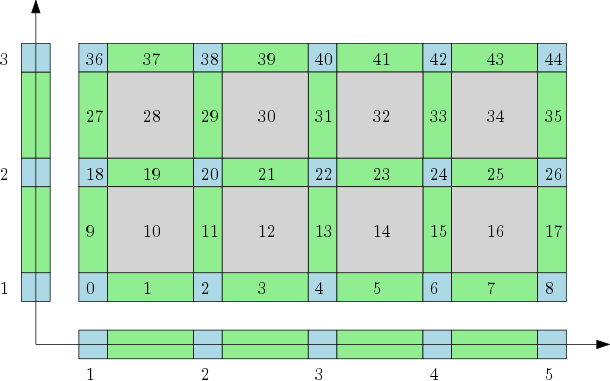The cubical complex represents a grid as a cell complex with cells of all dimensions. Author Pawel Dlotko Since GUDHI 2.0.0 License MIT

Simplicial complexes¶

Simplex tree¶The simplex tree is an efficient and flexible data structure for representing general (filtered) simplicial complexes. The data structure is described in  Author Clément Maria Since GUDHI 2.0.0 License MIT

Filtrations and reconstructions¶

Alpha complex¶Alpha complex is a simplicial complex constructed from the finite cells of a Delaunay Triangulation. It has the same persistent homology as the Čech complex and is significantly smaller. Author Vincent Rouvreau Since GUDHI 2.0.0 License MIT (GPL v3) Requires Eigen $$\geq$$ 3.1.0 and CGAL $$\geq$$ 4.11.0

Rips complex¶The Vietoris-Rips complex is a simplicial complex built as the clique-complex of a proximity graph. We also provide sparse approximations, to speed-up the computation of persistent homology, and weighted versions, which are more robust to outliers. Authors Clément Maria, Pawel Dlotko, Vincent Rouvreau, Marc Glisse, Yuichi Ike Since GUDHI 2.0.0 License MIT

Witness complex¶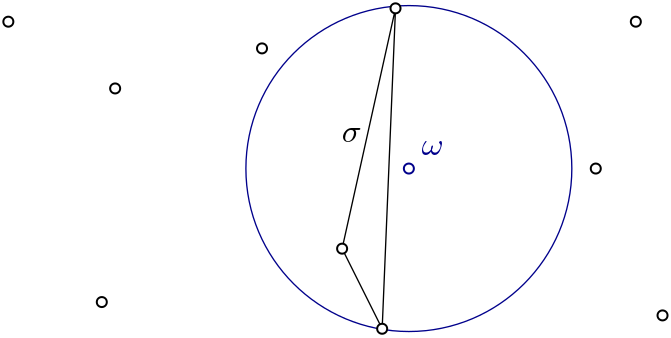Witness complex $$Wit(W,L)$$ is a simplicial complex defined on two sets of points in $$\mathbb{R}^D$$. The data structure is described in . Author Siargey Kachanovich Since GUDHI 2.0.0 License MIT (GPL v3 for Euclidean versions only) Requires Eigen $$\geq$$ 3.1.0 and CGAL $$\geq$$ 4.11.0 for Euclidean versions only

Cover complexes¶Nerves and Graph Induced Complexes are cover complexes, i.e. simplicial complexes that provably contain topological information about the input data. They can be computed with a cover of the data, that comes i.e. from the preimage of a family of intervals covering the image of a scalar-valued function defined on the data. Author Mathieu Carrière Since GUDHI 2.3.0 License MIT (GPL v3) Requires CGAL $$\geq$$ 4.11.0

Tangential complex¶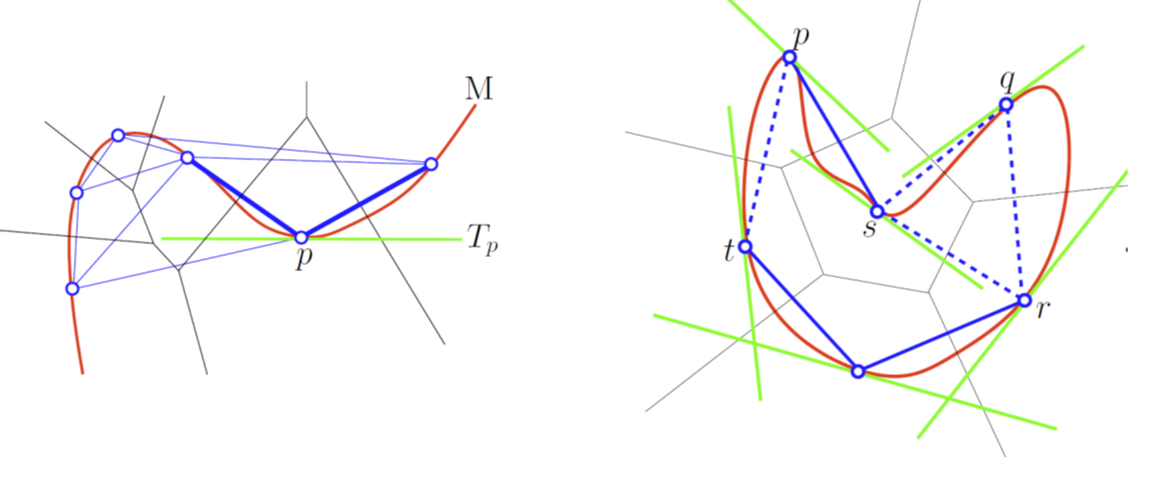A Tangential Delaunay complex is a simplicial complex designed to reconstruct a $$k$$-dimensional manifold embedded in $$d$$-dimensional Euclidean space. The input is a point sample coming from an unknown manifold. The running time depends only linearly on the extrinsic dimension $$d$$ and exponentially on the intrinsic dimension $$k$$. Author Clément Jamin Since GUDHI 2.0.0 License MIT (GPL v3) Requires Eigen $$\geq$$ 3.1.0 and CGAL $$\geq$$ 4.11.0

Topological descriptors computation¶

Persistent cohomology¶Rips Persistent Cohomology on a 3D Torus¶ The theory of homology consists in attaching to a topological space a sequence of (homology) groups, capturing global topological features like connected components, holes, cavities, etc. Persistent homology studies the evolution – birth, life and death – of these features when the topological space is changing. Computation of persistent cohomology using the algorithm of  and  and the Compressed Annotation Matrix implementation of . Author Clément Maria Since GUDHI 2.0.0 License MIT Please refer to each data structure that contains persistence feature for reference:

Topological descriptors tools¶

Bottleneck distance¶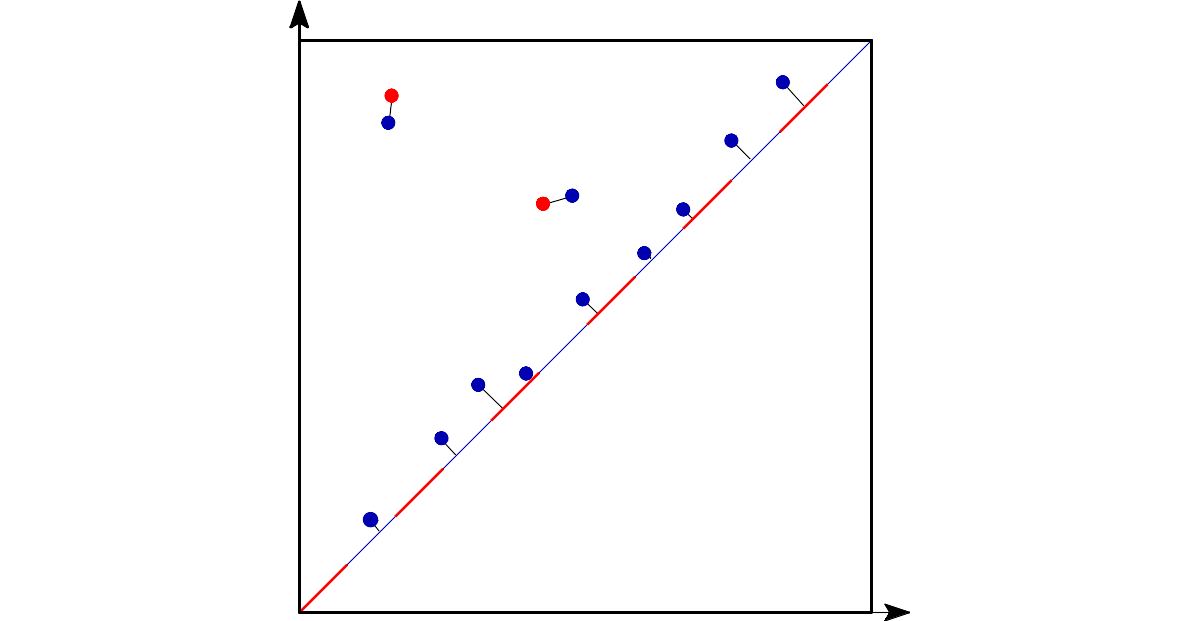Bottleneck distance is the length of the longest edge¶ Bottleneck distance measures the similarity between two persistence diagrams. It’s the shortest distance b for which there exists a perfect matching between the points of the two diagrams (+ all the diagonal points) such that any couple of matched points are at distance at most b, where the distance between points is the sup norm in $$\mathbb{R}^2$$. Author François Godi Since GUDHI 2.0.0 License MIT (GPL v3) Requires CGAL $$\geq$$ 4.11.0

Wasserstein distance¶The q-Wasserstein distance measures the similarity between two persistence diagrams using the sum of all edges lengths (instead of the maximum). It allows to define sophisticated objects such as barycenters of a family of persistence diagrams. Author Theo Lacombe, Marc Glisse Since GUDHI 3.1.0 License MIT, BSD-3-Clause

Persistence representations¶Vectorizations, distances and kernels that work on persistence diagrams, compatible with scikit-learn. Author Mathieu Carrière, Martin Royer Since GUDHI 3.1.0 License MIT Requires Scikit-learn

Persistence graphical tools¶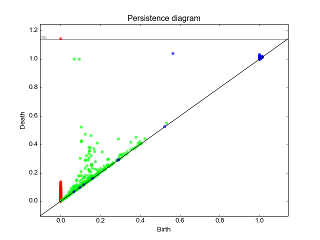These graphical tools comes on top of persistence results and allows the user to display easily persistence barcode, diagram or density. Note that these functions return the matplotlib axis, allowing for further modifications (title, aspect, etc.) Author Vincent Rouvreau, Theo Lacombe Since GUDHI 2.0.0 License MIT Requires Matplotlib

Point cloud utilities¶

 $$(x_1, x_2, \ldots, x_d)$$ $$(y_1, y_2, \ldots, y_d)$$ Utilities to process point clouds: read from file, subsample, find neighbors, embed time series in higher dimension, estimate a density, etc. Authors Vincent Rouvreau, Marc Glisse, Masatoshi Takenouchi Since GUDHI 2.0.0 License MIT (GPL v3, BSD-3-Clause, Apache-2.0)

Clustering¶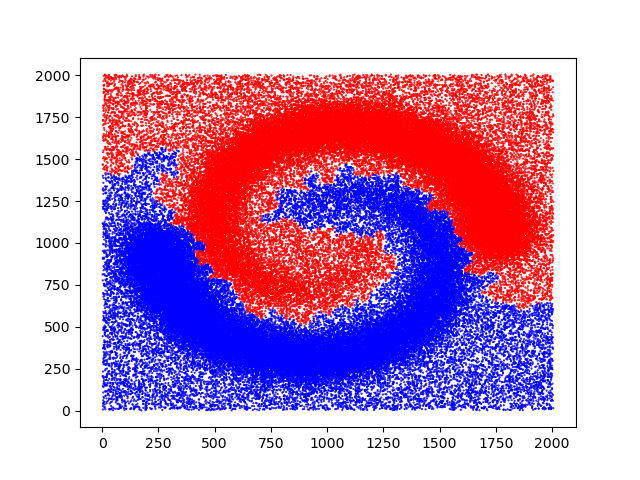Clustering tools. Author Marc Glisse Since GUDHI 3.3.0 License MIT

Datasets generators¶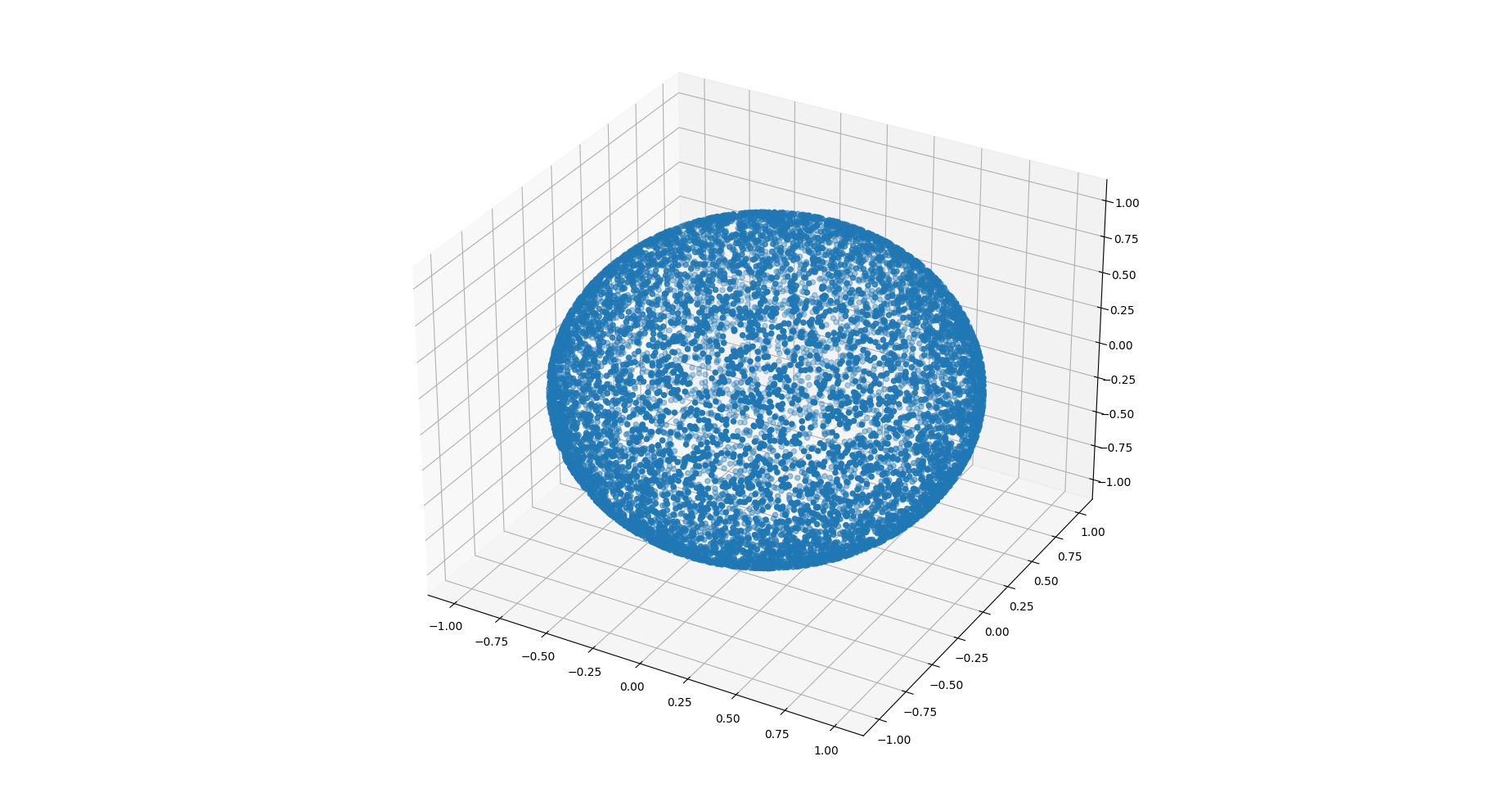Datasets generators (points). Authors Hind Montassif Since GUDHI 3.5.0 License MIT (LGPL v3) Requires CGAL